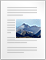# Quick Vector Algebra Summary

[latexpage]

Here in this post we will go through a quick recap of vector algebra keeping in mind that reader already had detail knowledge and problem solving skills related to the topic being discussed. Here we are briefing Vector Algebra because concepts of electrostatics , electromagnetism and many more physical phenomenon can best be conveniently expressed using this tool.

A vector is a quantity that requires both a magnitude (= 0) and a direction in space it can be represented by an arrow in space for example electrostatic force, electrostatic field etc. In symbolic form we will represent vectors by bold letters. In component form vector A is written as

$A=iA_{x}+jA_{y}+kA_{z}$

Two vectors A and B can be added together to give another resultant vector C.
C = A + B

SUBTRACTION OF VECTORS
Two vectors A and B can be subtracted to give another resultant vector D.
D = A – B = A + (-B)

SCALAR MULTIPLICATION OF VECTOR
When we multiply any vector A with any scalar quantity ‘n’ then it’s direction remains unchanged and magnitude gets multiplied by ‘n’. Thus,
n(A) = nA
Scalar multiplication of vectors is distributive i.e.,
n(A + B) = nA +nB

DOT PRODUCT OF VECTORS
Dot product of two vectors A and B is defined as the product of the magnitudes of vectors A and B and the cosine of the angle between them when both te vectors are placed tail to tail. Dot product is represented as A.B thus,
$\mathbf{A}.\mathbf{B}=|\mathbf{A}||\mathbf{B}|cos\theta$
where $\theta$ is the angle between two vectors.
Result of dot product of two vectors is a scalar quantity.
Dot product is commutative : A.B = B.A
Dot product is distributive : A . (B+C) = A.+ A.C also $\mathbf{A}.\mathbf{A}=|\mathbf{A}|^2$

CROSS PRODUCT OF TWO VECTORS
Cross product or vector product of two vectors A and B is defined as
$\mathbf{A}\times \mathbf{B}=|\mathbf{A}||\mathbf{B}|sin\theta\hat{n}$
where $\hat{n}$ is the unit vector pointing in the direction perpandicular to the plane of both A and B. Result of vector product is also a vector quantity.
Cross product is distributive i.e., A x (B + C) = (A x B) + (A x C) but not commutative and the cross product of two parallel vectors is zero.Vector algebra Synopsis (118.6 KiB)

### Search Our Website

Subscribe
Notify of

This site uses Akismet to reduce spam. Learn how your comment data is processed.

1 Comment
Inline Feedbacks